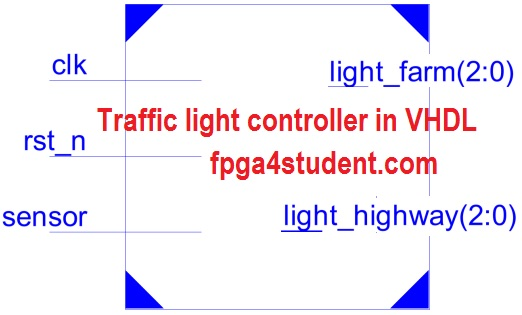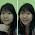# VHDL code for Traffic light controller

## A VHDL code for a traffic light controller on FPGA is presented. The traffic light controller in VHDL is used for an intersection between highway and farm way.

#### There is a sensor in the farm way side to detect if there is any vehicle on the farm way. If vehicles are detected on the farm way, traffic light on the high way turns to YELLOW, then RED so that the vehicles from the farm way can cross the high way. Otherwise, the traffic light on the high way is always GREEN and traffic light on the farm way is always RED. The time period is 3 seconds for the YELLOW light and 10 seconds for the RED light.### VHDL code for traffic light controller:

```-- fpga4student.com FPGA projects, VHDL projects, Verilog projects
-- VHDL project: VHDL code for traffic light controller
library IEEE;
use IEEE.STD_LOGIC_1164.ALL;
use IEEE.STD_LOGIC_UNSIGNED.ALL;
-- Traffic ligh system for a intersection between highway and farm way
-- There is a sensor on the farm way side, when there are vehicles,
-- Traffic light turns to YELLOW, then GREEN to let the vehicles cross the highway
-- Otherwise, always green light on Highway and Red light on farm way
entity traffic_light_controller is
port ( sensor  : in STD_LOGIC; -- Sensor
clk  : in STD_LOGIC; -- clock
rst_n: in STD_LOGIC; -- reset active low
light_highway  : out STD_LOGIC_VECTOR(2 downto 0); -- light outputs of high way
light_farm:    out STD_LOGIC_VECTOR(2 downto 0)-- light outputs of farm way
--RED_YELLOW_GREEN
);
end traffic_light_controller;
architecture traffic_light of traffic_light_controller is
signal counter_1s: std_logic_vector(27 downto 0):= x"0000000";
signal delay_count:std_logic_vector(3 downto 0):= x"0";
signal delay_10s, delay_3s_F,delay_3s_H, RED_LIGHT_ENABLE, YELLOW_LIGHT1_ENABLE,YELLOW_LIGHT2_ENABLE: std_logic:='0';
signal clk_1s_enable: std_logic; -- 1s clock enable
type FSM_States is (HGRE_FRED, HYEL_FRED, HRED_FGRE, HRED_FYEL);
-- HGRE_FRED : Highway green and farm red
-- HYEL_FRED : Highway yellow and farm red
-- HRED_FGRE : Highway red and farm green
-- HRED_FYEL : Highway red and farm yellow
signal current_state, next_state: FSM_States;
begin
-- next state FSM sequential logic
process(clk,rst_n)
begin
if(rst_n='0') then
current_state <= HGRE_FRED;
elsif(rising_edge(clk)) then
current_state <= next_state;
end if;
end process;
-- FSM combinational logic
process(current_state,sensor,delay_3s_F,delay_3s_H,delay_10s)
begin
case current_state is
when HGRE_FRED => -- When Green light on Highway and Red light on Farm way
RED_LIGHT_ENABLE <= '0';-- disable RED light delay counting
YELLOW_LIGHT1_ENABLE <= '0';-- disable YELLOW light Highway delay counting
YELLOW_LIGHT2_ENABLE <= '0';-- disable YELLOW light Farmway delay counting
light_highway <= "001"; -- Green light on Highway
light_farm <= "100"; -- Red light on Farm way
if(sensor = '1') then -- if vehicle is detected on farm way by sensors
next_state <= HYEL_FRED;
-- High way turns to Yellow light
else
next_state <= HGRE_FRED;
-- Otherwise, remains GREEN ON highway and RED on Farm way
end if;
when HYEL_FRED => -- When Yellow light on Highway and Red light on Farm way
light_highway <= "010";-- Yellow light on Highway
light_farm <= "100";-- Red light on Farm way
RED_LIGHT_ENABLE <= '0';-- disable RED light delay counting
YELLOW_LIGHT1_ENABLE <= '1';-- enable YELLOW light Highway delay counting
YELLOW_LIGHT2_ENABLE <= '0';-- disable YELLOW light Farmway delay counting
if(delay_3s_H='1') then
-- if Yellow light delay counts to 3s,
-- turn Highway to RED,
-- Farm way to green light
next_state <= HRED_FGRE;
else
next_state <= HYEL_FRED;
-- Remains Yellow on highway and Red on Farm way
-- if Yellow light not yet in 3s
end if;
when HRED_FGRE =>
light_highway <= "100";-- RED light on Highway
light_farm <= "001";-- GREEN light on Farm way
RED_LIGHT_ENABLE <= '1';-- enable RED light delay counting
YELLOW_LIGHT1_ENABLE <= '0';-- disable YELLOW light Highway delay counting
YELLOW_LIGHT2_ENABLE <= '0';-- disable YELLOW light Farmway delay counting
if(delay_10s='1') then
-- if RED light on highway is 10s, Farm way turns to Yellow
next_state <= HRED_FYEL;
else
next_state <= HRED_FGRE;
-- Remains if delay counts for RED light on highway not enough 10s
end if;
when HRED_FYEL =>
light_highway <= "100";-- RED light on Highway
light_farm <= "010";-- Yellow light on Farm way
RED_LIGHT_ENABLE <= '0'; -- disable RED light delay counting
YELLOW_LIGHT1_ENABLE <= '0';-- disable YELLOW light Highway delay counting
YELLOW_LIGHT2_ENABLE <= '1';-- enable YELLOW light Farmway delay counting
if(delay_3s_F='1') then
-- if delay for Yellow light is 3s,
-- turn highway to GREEN light
-- Farm way to RED Light
next_state <= HGRE_FRED;
else
next_state <= HRED_FYEL;
-- if not enough 3s, remain the same state
end if;
when others => next_state <= HGRE_FRED; -- Green on highway, red on farm way
end case;
end process;
-- Delay counts for Yellow and RED light
process(clk)
begin
if(rising_edge(clk)) then
if(clk_1s_enable='1') then
if(RED_LIGHT_ENABLE='1' or YELLOW_LIGHT1_ENABLE='1' or YELLOW_LIGHT2_ENABLE='1') then
delay_count <= delay_count + x"1";
if((delay_count = x"9") and RED_LIGHT_ENABLE ='1') then
delay_10s <= '1';
delay_3s_H <= '0';
delay_3s_F <= '0';
delay_count <= x"0";
elsif((delay_count = x"2") and YELLOW_LIGHT1_ENABLE= '1') then
delay_10s <= '0';
delay_3s_H <= '1';
delay_3s_F <= '0';
delay_count <= x"0";
elsif((delay_count = x"2") and YELLOW_LIGHT2_ENABLE= '1') then
delay_10s <= '0';
delay_3s_H <= '0';
delay_3s_F <= '1';
delay_count <= x"0";
else
delay_10s <= '0';
delay_3s_H <= '0';
delay_3s_F <= '0';
end if;
end if;
end if;
end if;
end process;
-- create delay 1s
process(clk)
begin
if(rising_edge(clk)) then
counter_1s <= counter_1s + x"0000001";
if(counter_1s >= x"0000003") then -- x"0004" is for simulation
-- change to x"2FAF080" for 50 MHz clock running real FPGA
counter_1s <= x"0000000";
end if;
end if;
end process;
clk_1s_enable <= '1' when counter_1s = x"0003" else '0'; -- x"0002" is for simulation
-- x"2FAF080" for 50Mhz clock on FPGA
end traffic_light;```

### VHDL Testbench code for traffic light controller:

```-- fpga4student.com FPGA projects, VHDL projects, Verilog projects
-- VHDL project: VHDL code for traffic light controller
LIBRARY ieee;
USE ieee.std_logic_1164.ALL;
-- Testbench VHDL code for traffic light controller
ENTITY tb_traffic_light_controller IS
END tb_traffic_light_controller;

ARCHITECTURE behavior OF tb_traffic_light_controller IS
-- Component Declaration for the traffic light controller
COMPONENT traffic_light_controller
PORT(
sensor : IN  std_logic;
clk : IN  std_logic;
rst_n : IN  std_logic;
light_highway : OUT  std_logic_vector(2 downto 0);
light_farm : OUT  std_logic_vector(2 downto 0)
);
END COMPONENT;
signal sensor : std_logic := '0';
signal clk : std_logic := '0';
signal rst_n : std_logic := '0';
--Outputs
signal light_highway : std_logic_vector(2 downto 0);
signal light_farm : std_logic_vector(2 downto 0);
constant clk_period : time := 10 ns;
BEGIN
-- Instantiate the traffic light controller
trafficlightcontroller : traffic_light_controller PORT MAP (
sensor => sensor,
clk => clk,
rst_n => rst_n,
light_highway => light_highway,
light_farm => light_farm
);
-- Clock process definitions
clk_process :process
begin
clk <= '0';
wait for clk_period/2;
clk <= '1';
wait for clk_period/2;
end process;
stim_proc: process
begin
rst_n <= '0';
sensor <= '0';
wait for clk_period*10;
rst_n <= '1';
wait for clk_period*20;
sensor <= '1';
wait for clk_period*100;
sensor <= '0';
wait;
end process;

END;```

#### Simulation waveform for the traffic light controller in VHDL:1.Hello. I have tried this code using the 50 MHZ clock (Y1) but it couldn't work. Can u help me solve this ? Many Thanks

1.Did you synthesize the code? What did the tool say after synthesis? Was the timing met?

2.Hi.I have the same problem .Can you help me solve this problem

1.Did you change the clock divisor as mentioned in the code when 50Mhz clock is used?

2.Thank you :)

3.Hi sir.Can you help me to display delay in seven-segment from this program

1.Here:
http://www.fpga4student.com/2017/09/seven-segment-led-display-controller-basys3-fpga.html
http://www.fpga4student.com/2017/09/vhdl-code-for-seven-segment-display.html

4.hi! How can I use a segment7 to show the time left for RED light and GREEN light in the vhdl code? Can u help me?

1.Here:
http://www.fpga4student.com/2017/09/seven-segment-led-display-controller-basys3-fpga.html
http://www.fpga4student.com/2017/09/vhdl-code-for-seven-segment-display.html

5.Hello,

How could you write this same code without using "after" , "wait for" or state machines?

I think I can do it with counters, using processes, but I don't know how...

6.Hello,
how could you write this same code without using "after" , "wait for", or state machines?

I think I can use counters, using processes, but I don't know how...

7.which type of fpga you used here?

8.which type of fpga used here?

1.You can use any FPGA to run this simple project.

9.This project for 2way traffic light or 4way??

10.11.I am new to VHDL coding but
Could you use only one yellow_light_enable?
Rather than using two that do the same thing?

1.it's up to you. Do whatever you want as long as simulation works.

12.hello sir,can u put a detailed explanation of this code

13.How do we put this code into the FPGA Hardware?

14.Hi, what are the electronic materials needed in order to create this project? Thankss!